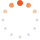# Tistory

티스토리 메뉴 펼치기

티스토리 메뉴 펼치기 댓글수0

프로그래밍/LeetCode

## [LeetCode][Python3] 5. Longest Palindromic Substring

snoopybox
댓글수0

Problem :

https://leetcode.com/problems/longest-palindromic-substring/

My Solution :

class Solution:
def longestPalindrome(self, s: str) -> str:
L, R = 0, len(s)

def expand(left, right):
while L <= left and right < R and s[left] == s[right]:
left -= 1
right += 1
return right - left - 1

ans = ''
for i in range(len(s)):
size = max(expand(i, i), expand(i, i+1))
if size > len(ans):
ans = s[i-(size-1)//2:i+1+size//2]
return ans### 이 블로그 인기 글

맨위로

https://www.snoopybox.co.kr/2008